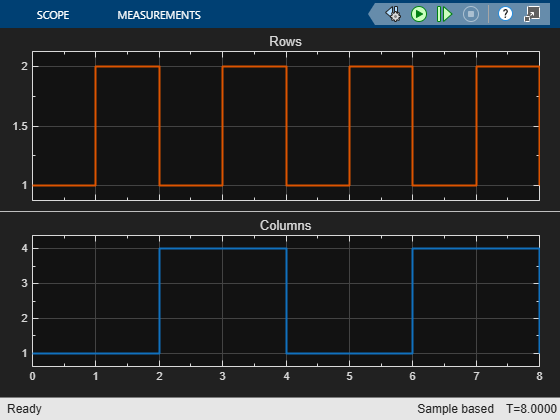# Compute Output Based on Size of Input Signal

This example shows how to define variable-size output data in Stateflow® charts. In this model, the chart `VarSizeSignalSource` generates a variable-size signal. The chart `SizeBasedProcessing` analyzes this signal and produces a related variable-size signal. Display blocks show the values and size of the two signals.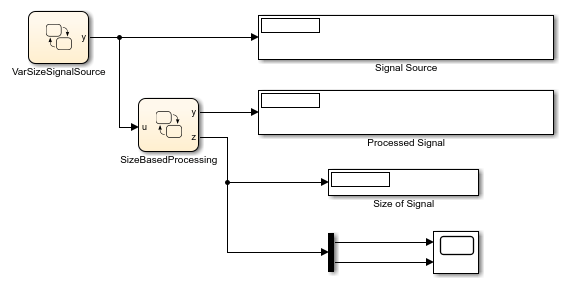For more information on variable-size data, see Declare Variable-Size Data in Stateflow Charts.

### Generate Variable-Size Output Data

The Stateflow chart `VarSizeSignalSource` uses MATLAB® as the action language. The temporal logic in this chart triggers the transitions between four states. Each state generates an output value with a different size: a scalar, a two-element column vector, a four-element row vector, and a 2-by-4 matrix.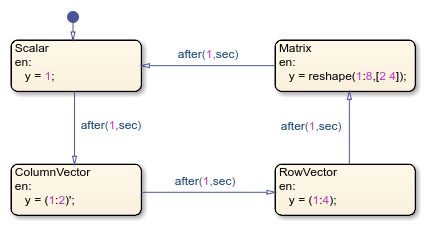The chart behaves like a source block. It has no input and one variable-size output, `y`. For variable-size outputs, you must explicitly specify the upper bounds for each dimension. In this case, the Variable size property for `y` is enabled and its Size property is set to `[2 4]`, the maximum size for the signal.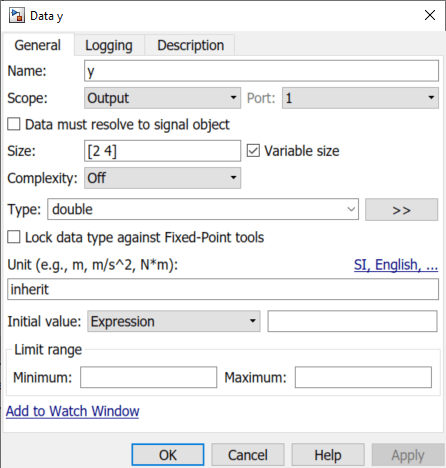In charts that use MATLAB as the action language, state and transition actions can read and write directly to variable-size data. For example, the entry actions of the states in this chart explicitly compute the value of `y`.

### Process Variable-Size Input Data

The Stateflow chart `SizeBasedProcessing` uses C as the action language. These charts can exchange variable-size data with other charts and blocks in the model. However, state and transition actions in C charts cannot read from or write to variable-size data directly. All computations involving variable-size data must occur in MATLAB functions, Simulink® functions, and truth tables that use MATLAB as the action language.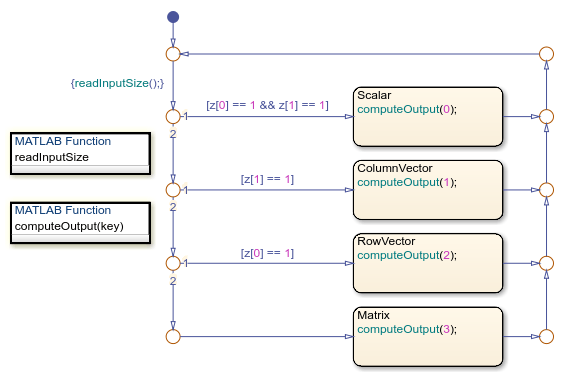This chart has two variable-size data objects:

• Input `u` is the variable-size signal generated by the `VarSizeSignalSource` chart. Because the chart property Support variable-size arrays is enabled, this chart accepts variable-size input data from other blocks in the Simulink model. The Size property for `u` is set to `-1` to indicate that the chart inherits the maximum size for this input from the output in chart `VarSizeSignalSource`.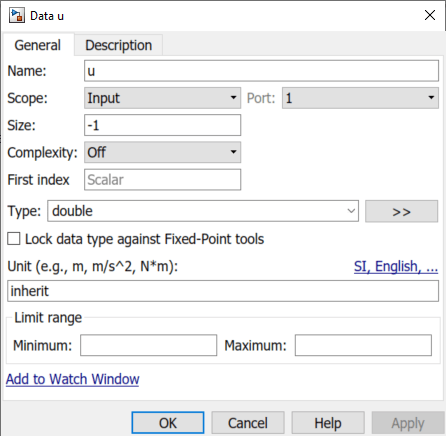• Output `y` is a variable-size signal whose size and value depends on whether `u` is a scalar, a vector, or a matrix. The Size property for `u` is set to `[2 4]`, the maximum size for the signal.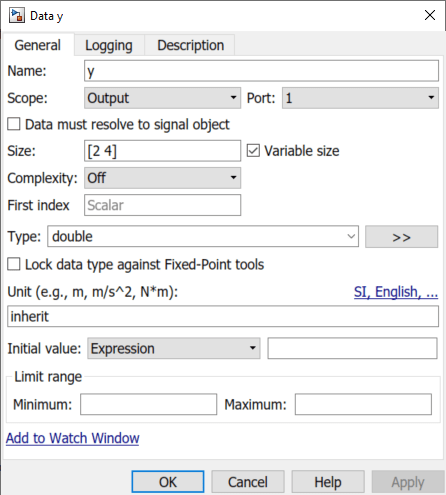Because this chart uses C as the action language, the names of the variable-size data do not appear in the state actions or transition logic. Instead, the transition logic in the chart calls the MATLAB function `readInputSize` to determine the size of the input `u`. Similarly, the actions in the states call the MATLAB function `computeOutput` to produce values of various size for the variable-size output `y`. Because MATLAB functions can access chart-level data directly, you do not have to pass the variable-size data as inputs or outputs to these functions.

### Determine Size of Input

The MATLAB function `isScalarInput` determines the size of the chart input `u`. This signal, which is generated by chart `VarSizeSignalSource`, can be a scalar, a 2-by-1 column vector, a 1-by-4 row vector, or a 2-by-4 matrix. The function stores the dimensions of `u` as the chart-level output `z`.

```function readInputSize %#codegen z = size(u); end ```

### Produce Variable-Size Output

The MATLAB function `computeOutput` computes the value of the chart output `y` based on the size and value of the chart input `u`.

• If `u` is a scalar, the function assigns to `y` a scalar value of zero.

• If `u` is a column vector, the function computes of the sine of each of its elements and stores them in `y`.

• If `u` is a row vector, the function computes of the cosine of each of its elements and stores them in `y`.

• If `u` is a matrix, the function computes of the square root of each of its elements and stores them in `y`.

In each case, the value of the output `y` has the same size as the input `u`.

```function computeOutput(key) %#codegen switch key case 0 % scalar y = 0; case 1 % column vector y = sin(u); case 2 % row vector y = cos(u); case 3 % matrix y = sqrt(u); end end ```

### Simulate the Model

The tabs located above the Explorer Bar enable you to switch between the Simulink model and the two Stateflow charts. During simulation:

• The chart animations show the active state for each chart cycling between the `Scalar`, `ColumnVector`, `RowVector`, and `VectorPartial`, and `Matrix` states.

• In the Simulink model, the display blocks `Signal Source` and `Processed Signal` periodically show between one and eight values for the variable-size signals.

• The display block `Size of Signal` and the Scope block show the number of rows and columns in the variable-size signals.